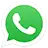## POROSITY (Non-Equilibrium, Thermal models in Porous Media)

Non-Equilibrium Thermal models in porous media To explain this investigation about the porosity, at first, we must say that there are two thermal models for the porous medium: The first model assumes the thermal balance between the fluid and the…

## POROSITY (Viscous and Inertial Resistances Coefficients, Power-law Model, and Prosthesis Effect on Blood)

Porosity Obtaining viscous and inertial resistance coefficients We must have a laboratory sample of our porous (Porosity) medium  to obtain these coefficients. The experiment should calculate the pressure drop across the porous region at different velocities. Then, we obtain a quadratic…

## POROSITY (Viscous & Inertial Resistances and Heterogeneous Porous Medium)

Porosity Introduction This is a porosity introduction. A porous medium is a material in which all the volume is not solid and has void holes. However, if this medium is in front of the motion of the fluid, the fluid…Call On WhatsApp